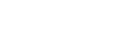# Problem: A change in temperature from 10°C to 20°C is found to double the rate of a particular chemical reaction. How did the change in temperature affect the reacting molecules?(A) The average velocity of the molecules doubled(B) The average energy of the molecules doubled(C) The number of collisions per second doubled(D) The number of molecules above the reaction energy threshold doubled.

###### FREE Expert Solution
86% (342 ratings)
###### Problem Details

A change in temperature from 10°C to 20°C is found to double the rate of a particular chemical reaction. How did the change in temperature affect the reacting molecules?

(A) The average velocity of the molecules doubled

(B) The average energy of the molecules doubled

(C) The number of collisions per second doubled

(D) The number of molecules above the reaction energy threshold doubled.What scientific concept do you need to know in order to solve this problem?

Our tutors have indicated that to solve this problem you will need to apply the Rate of Reaction concept. You can view video lessons to learn Rate of Reaction. Or if you need more Rate of Reaction practice, you can also practice Rate of Reaction practice problems.

What is the difficulty of this problem?

Our tutors rated the difficulty ofA change in temperature from 10°C to 20°C is found to double...as high difficulty.

How long does this problem take to solve?

Our expert Chemistry tutor, Dasha took 5 minutes and 19 seconds to solve this problem. You can follow their steps in the video explanation above.

What professor is this problem relevant for?

Based on our data, we think this problem is relevant for Professor Khakimova's class at BELLEVUE COLLEGE.